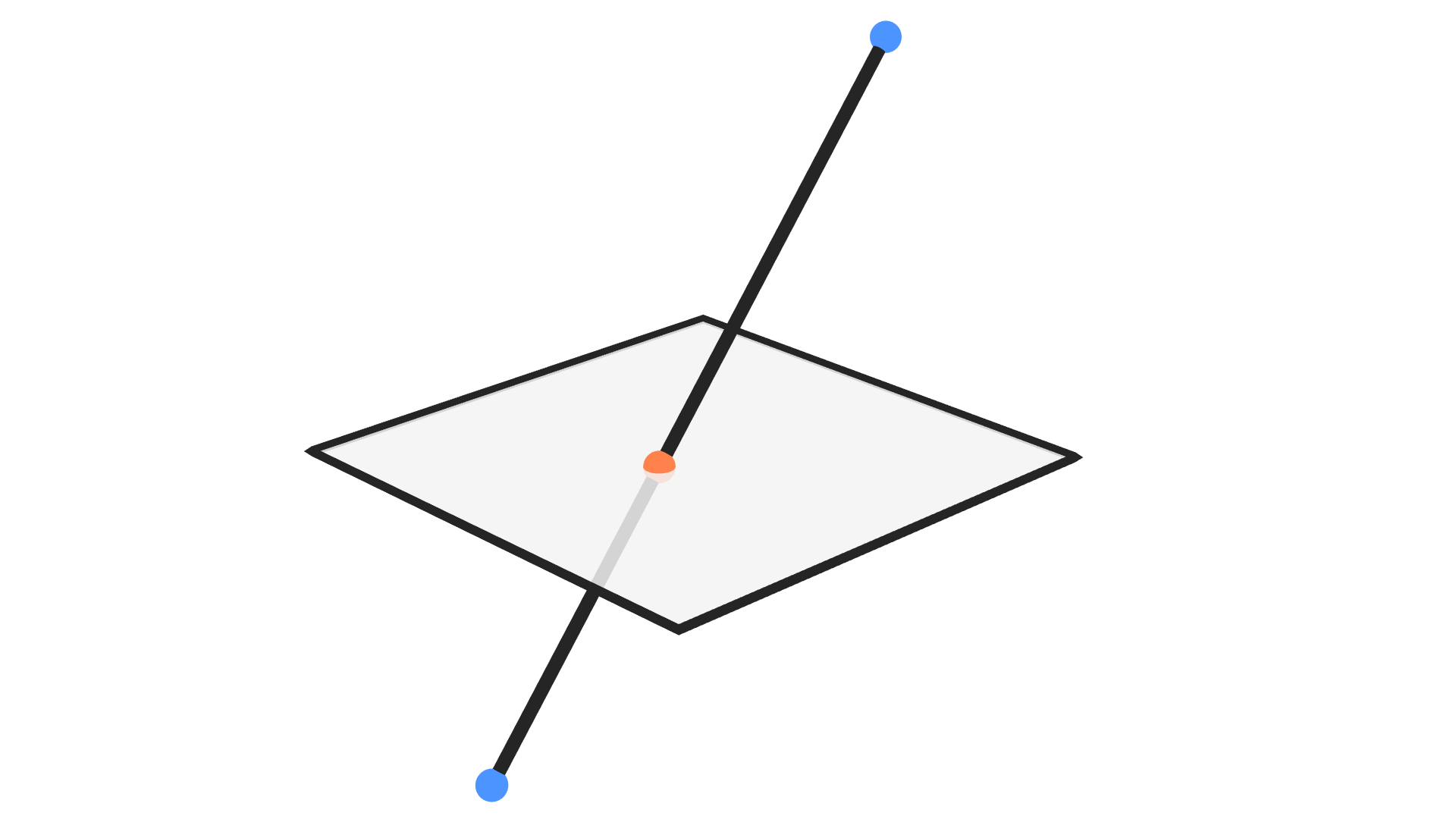# Intersect Line Plane

## Description

This node returns the location of the point of intersection of the input line with the input plane.

## IllustrationBlue points are the input points and orange point is the intersection with the input plane.

## Inputs

• Line Start - The location of the starting point of the line.
• Line End - The location of the ending point of the line.
• Plane Point - A point on the required plane.
• Plane Normal - A unit vector that represent the normal of the required plane.

## Outputs

• Intersection - The location of the point of intersection of the input line and input plane.
• Is Valid - A boolean which is True if an intersection was found and False otherwise. The intersection will not be found only when the line is perpendicular on the normal of the plane.